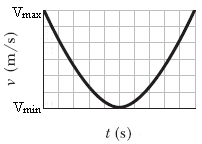# Projectile Motion Analysis

• cahp19

## Homework Statement

A golf ball is struck at ground level. The speed of the golf ball as a function of time is shown in the figure below, where t = 0 at the instant the ball is struck. The graph is marked in increments of 0.55 s along the time axis, and vmin = 18.92 m/s and vmax = 32.93 m/s. (Values in figure do not necessarily match values in problem).

This graph is then provided:a) How far does the golf ball travel horizontally before returning to ground level?
b) What is the maximum height above the ground level attained by the ball?

## Homework Equations

I'm not sure, because each applicable equation I find has the need for a theta in it.

## The Attempt at a Solution

I can't even find a working equation.

Thanks a lot!

What values can you pick out from the given information? List them.
(Hint: some may require looking for points of interest on the graph and measuring/deducing values).

What values can you pick out from the given information? List them.
(Hint: some may require looking for points of interest on the graph and measuring/deducing values).

The points that I am able to get from the graph are:
t(0)= 32.93 m/s
t(2.75)= 18.92 m/s
t(5.5)= 32.93 m/s

You also know the total time the ball is in flight.

Plus what does the value of Vmin=18.92 m/s tell you about the vertical and/or horizontal velocity at that time?